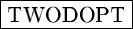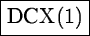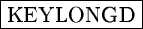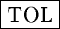Next: Possible Graphical Verifications Up: Getting the Dispersion Solution Previous: Line Identification

### Determination of the Dispersion Coefficients

The command CALIBRATE/LONG determines the dispersion relation for each row of the spectrum. In addition, a bivariate dispersion relation is computed if the keywordis set to YES, as in:

Midas...> SET/LONG TWODOPT=YES
Midas...> CALIBRATE/LONG

The command CALIBRATE/LONG determines row-by-row polynomial solutions of degreestored in the table coerbr.tbl and fits a 2-D polynomial of degreeto the lines with entries in columns :X, :Y, and :WAVE of table line.tbl. The coefficients of the 2D polynomial are stored in the keyword. The program performs a final oulier rejection which eliminates all lines with residuals larger thanpixels (if TOL is positive). However the minimum number of lines in any given row will be+2. If this number cannot be obtained, a polynomial of lower order will be fitted. Three more columns are added to the table line.tbl:

 :WAVE identified wavelength :WAVEC wavelength computed from polynomial fit :RESIDUAL (= :WAVEC - :WAVE) Residual of polynomial fit from the tabulated wavelength)

Note
For a proper calibration, images should preferably have positive step descriptors and the wavelength should increase from left to right.Next: Possible Graphical Verifications Up: Getting the Dispersion Solution Previous: Line Identification
Petra Nass
1999-06-15